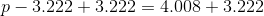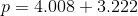## Example Questions

### Example Question #1 : Decimals

Solve for: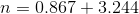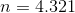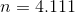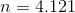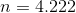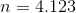Explanation:

To add decimals, simply treat them like you would any other number.  Any time two of the digits in a particular place (i.e. tenths, hundredths, thousandths) add up to more than ten, you have to carry the one to the next greatest column.  Therefore: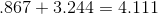So.

### Example Question #2 : Decimals

Solve for: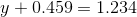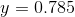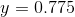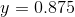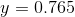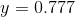Explanation:

To solve this problem, subtract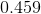from both sides of the eqution,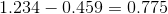Therefore,.

If you're having trouble subtracting the decimal, mutliply both numbers byfollowed by a number of zeroes equal to the number of decimal places.  Then subtract, then divide both numbers by the number you multiplied them by.

### Example Question #3 : Decimals

Solve for: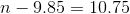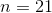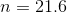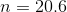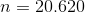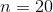Explanation:

To solve, first add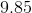to both sides of the equation: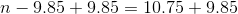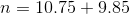### Example Question #4 : Decimals

Solve for: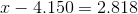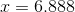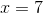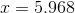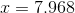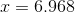Explanation:

To solve, you need to do some algebra:Isolate x by adding the 4.150 to both sides of the equation.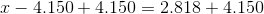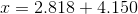Then add the decimals.  If you have trouble adding decimals, an effective method is to place one decimal over the other, and add the digits one at a time.  Remember to carry every time the digits in a given place add up to more than.### Example Question #5 : Decimals

Solve for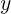: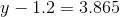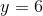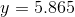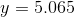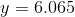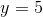Explanation:

To solve for, first add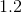to both sides of the equation, so that you isolate the variable: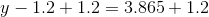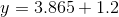Then, add your decimals, and remember that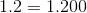.### Example Question #6 : Decimals

Solve for: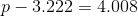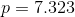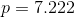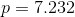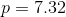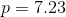Explanation:

To solve, first add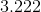to both sides of your equation, so you isolate the variable: